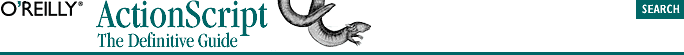home | O'Reilly's CD bookshelfs | FreeBSD | Linux | Cisco | Cisco Exam## 5.4. The Equality and Inequality Operators

`operand1 == operand2`

where operand1 and operand2 may be any valid expression. The equality operator can compare operands of any type. When operand1 and operand2 are equal, the expression returns the Boolean value true; when they differ, it returns the Boolean value false. For example:

```var x = 2;
x == 1     // false
x == 2     // true```

#### TIP

The equality operator is created using two equal signs in a row (==). It determines whether two expressions are equal and should not be confused with the assignment operator (=) which is used to assign a variable a new value.

Consider this example:

```if (x = 5) {
trace ("x is equal to 5")
}```

The preceding example does not check whether x equals 5. Instead, it sets x equal to 5. The proper expression is as follows:

```// Use == instead of =
if (x == 5) {
trace ("x is equal to 5")
}```

### 5.4.4. The Inequality Operator

`operand1 != operand2`

For example:

```var a = 5;
var b = 6;
var c = 6;
a != b  // true
b != c  // false```

The inequality operator follows the same type conversion rules as the equality operator and always yields the opposite result, including when using NaN as one operand:

```NaN != 7    // true
NaN != NaN  // true!```

In some languages, including Flash4 ActionScript, the <> operator is used as the inequality operator. See also the NOT operator (!) discussed later.